﻿ 温度依赖功能梯度Timoshenko梁的弹塑性屈曲

# 温度依赖功能梯度Timoshenko梁的弹塑性屈曲Elastoplastic Buckling of Temperature Dependence Functionally Graded Timoshenko Beam

Abstract: The elastoplastic buckling characteristics of functionally graded Timoshenko beams in varying temperature field are studied by considering the temperature dependence of material parameters. The elastoplastic material parameters of functionally graded materials are simulated by TTO model and the elastoplastic constitutive equation is established by linear strengthening model. By using the symplectic method in Hamilton system, the problem is transformed into solving canonical equations. The critical load and buckling mode correspond to the symplectic eigenvalue and eigen solution of the canonical equations, and are obtained by accurate analytical solution. At the same time, the elastoplastic interface is obtained by combining the yield condition. Finally, the influence of gradient parameters, geometric parameters and boundary conditions on the buckling load and elastoplastic deformation interface of the beam is analyzed.

1. 引言

2. 控制方程及求解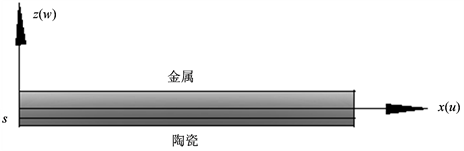Figure 1. Functionally graded Timoshenko beam

2.1. 等效物性参数

FGM考虑由陶瓷和金属两相材料制成，且假设其体积分数 ${V}_{\text{c}}$${V}_{\text{m}}$ 沿厚度方向以幂函数形式连续变化：

${V}_{\text{c}}\left(z\right)={\left(\frac{h-2z}{2h}\right)}^{n}$, ${V}_{\text{m}}\left(z\right)=1-{V}_{\text{c}}\left(z\right)$ (1)

${P}_{j}\left(T\right)={P}_{0}\left({P}_{-1}{T}^{-1}+1+{P}_{1}T+{P}_{2}{T}^{2}+{P}_{3}{T}^{3}\right)$ (2)

$E={E}_{\text{m}}{V}_{\text{m}}+{E}_{\text{c}}{V}_{\text{c}}$, $H={H}_{\text{m}}{V}_{\text{m}}+{E}_{\text{c}}{V}_{\text{c}}$, ${\sigma }_{\text{Y}}={\sigma }_{\text{Ym}}\left({V}_{\text{m}}+\frac{{E}_{\text{c}}}{{E}_{\text{m}}}{V}_{\text{c}}\right)$ (3)

2.2. 基本方程和边界条件

FGM梁的正应变 ${\epsilon }_{x}$ 和切应变 ${\gamma }_{xz}$ 基于Timoshenko梁理论建立：

${\epsilon }_{x}=\frac{\partial {u}_{0}}{\partial x}-z\frac{\partial \phi }{\partial x}$, ${\gamma }_{xz}={\gamma }_{xz}^{0}=\frac{\partial {w}_{0}}{\partial x}-\phi$ (4)

FGM的本构关系基于线性强化弹塑性模型建立，正应力 ${\sigma }_{x}$ 和切应力分别为 ：

${\sigma }_{x}=\left\{\begin{array}{l}E\left({\epsilon }_{x}-\alpha \Delta T\right)\text{}-\frac{h}{2}\le z (5a)

${\tau }_{xz}=G{\gamma }_{xz}$ (5b)

$\text{C}:{u}_{0}\text{=}{w}_{0}=\phi =0$, $\text{S}:{u}_{0}\text{=}{w}_{0}=M=0$.

2.3. 正则方程

$\stackrel{˙}{w}=\frac{\partial w}{\partial t}$${w}^{\prime }=\frac{\partial w}{\partial x}$$\stackrel{˙}{\phi }=\frac{\partial \phi }{\partial t}$${\phi }^{\prime }=\frac{\partial \phi }{\partial x}$，FGM梁的Lagrange密度函数可表示为：

$\begin{array}{l}\stackrel{¯}{L}=\stackrel{¯}{T}-\stackrel{¯}{V}-\stackrel{¯}{U}\\ \text{}\frac{1}{2}\stackrel{¯}{{I}_{0}}{\left(\stackrel{˙}{w}\right)}^{2}+\frac{1}{2}\stackrel{¯}{{I}_{1}}{\left(\stackrel{˙}{\phi }\right)}^{2}-\frac{1}{2}Q{\left({w}^{\prime }\right)}^{2}\\ \text{}-\frac{1}{2}{B}_{1}{\left({\phi }^{\prime }\right)}^{2}-\frac{1}{2}{B}_{2}\left({\phi }^{\prime }\right)-\frac{1}{2}{B}_{3}{\left({w}^{\prime }-\phi \right)}^{2}\end{array}$ (6)

$F=p\stackrel{˙}{q}-L=\frac{{p}_{1}^{2}}{2{I}_{0}}+\frac{{p}_{2}^{2}}{2{I}_{1}}+{A}_{1}{\left({W}^{\prime }\right)}^{2}+{\left({\varphi }^{\prime }\right)}^{2}+{A}_{2}\left({\varphi }^{\prime }\right)+{A}_{3}{\left({W}^{\prime }-\varphi \right)}^{2}$ (7)

$\stackrel{˙}{\psi }\equiv \left\{\begin{array}{c}\frac{\delta F}{\delta p}\\ -\frac{\delta F}{\delta q}\end{array}\right\}=\left\{\begin{array}{c}\frac{{p}_{1}}{{I}_{0}}\\ \frac{{p}_{2}}{{I}_{1}}\\ \left({A}_{1}+{A}_{3}\right)\frac{{\partial }^{2}{q}_{1}}{\partial {x}^{2}}-{A}_{3}\frac{\partial {q}_{2}}{\partial x}\\ \frac{{\partial }^{2}{q}_{2}}{\partial {x}^{2}}+{A}_{3}\left(\frac{\partial {q}_{1}}{\partial x}-{q}_{2}\right)\end{array}\right\}$ (8)

2.4. 方程的解析解

$\left({A}_{1}+{A}_{3}\right)\frac{{\partial }^{2}{q}_{1}}{\partial {x}^{2}}-{A}_{3}\frac{\partial {q}_{2}}{\partial x}=0$ (9a)

$\frac{{\partial }^{2}{q}_{2}}{\partial {x}^{2}}+{A}_{3}\left(\frac{\partial {q}_{1}}{\partial x}-{q}_{2}\right)=0$ (9b)

$W=C\left[{C}_{1}+{C}_{2}x-\frac{{C}_{3}\mathrm{cos}\left(\sqrt{\theta }x\right)}{\theta }-\frac{{C}_{4}\mathrm{sin}\left(\sqrt{\theta }x\right)}{\theta }\right]$ (10)

$2-2\mathrm{cos}\sqrt{\theta }-\sqrt{\theta }\mathrm{sin}\sqrt{\theta }=0$ (11a)

C-S边界为：

$-\left(\theta +1\right)\mathrm{cos}\sqrt{\theta }+\sqrt{\theta }\mathrm{sin}\sqrt{\theta }+1=0$ (11b)

${W}_{i}=C\left[\frac{1-\mathrm{cos}\sqrt{\theta }}{\mathrm{sin}\sqrt{\theta }}-\sqrt{\theta }x-\frac{\mathrm{sin}\sqrt{\theta }x}{\theta }+\frac{\left(\mathrm{cos}\sqrt{\theta }-1\right)\mathrm{cos}\sqrt{\theta }x}{\mathrm{sin}\left(\sqrt{\theta }\right)\theta }\right]$ (12a)

C-S边界为：

${W}_{i}=C\left[\mathrm{tan}\sqrt{\theta }-\sqrt{\theta }x-\frac{\mathrm{sin}\sqrt{\theta }x}{\theta }+\frac{\mathrm{sin}\left(\sqrt{\theta }\right)\mathrm{cos}\left(\sqrt{\theta }x\right)}{\mathrm{cos}\left(\sqrt{\theta }\right)\theta }\right]$ (12b)

FGM梁发生弹塑性屈曲，为了求解弹塑性变形的分界面到中面的距离 $s$，可利用屈服条件 $\sigma \left(s\right)={\sigma }_{Y}\left(s\right)$。将特征值 ${\theta }_{i}$ 依次回代，并通过数值解方程，可以得到临界屈曲载荷N和弹塑性交界面s。

3. 数值算例和讨论

3.1. 临界屈曲载荷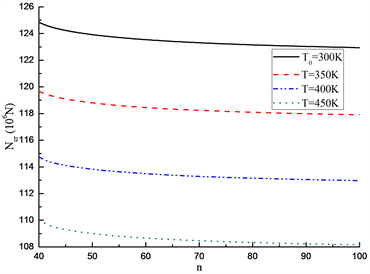(a) C-C边界(Clamped-Clamped boundary)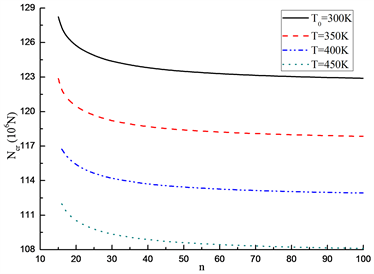(b) C-S边界(Clamped-Simply supported boundary)

Figure 2. Effect of volume fraction index n on critical load ${N}_{cr}$ ( $L=5$ )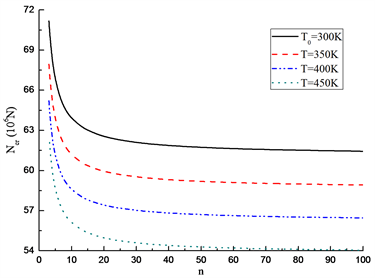(a) C-C边界(Clamped-Clamped boundary condition)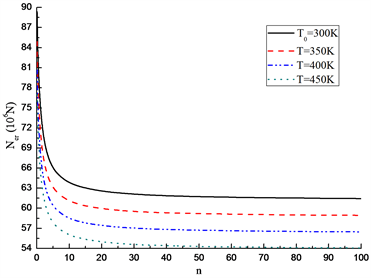(b) C-S边界(Clamped-Simply supported boundary)

Figure 3. Effect of volume fraction index n on critical load ${N}_{cr}$ ( $L=10$ )

3.2. 屈服界面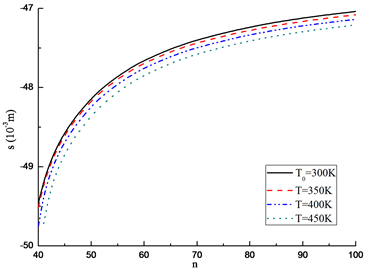(a) C-C边界(Clamped-Clamped boundary condition)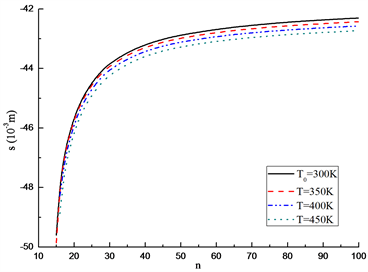(b) C-S边界(Clamped-Simply supported boundary)

Figure 4. Effect of volume fraction index n on elastoplastic interface s ( $L=5$ )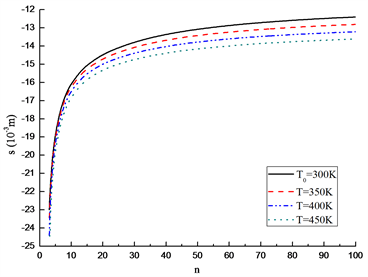(a) C-C边界(Clamped-Clamped boundary condition)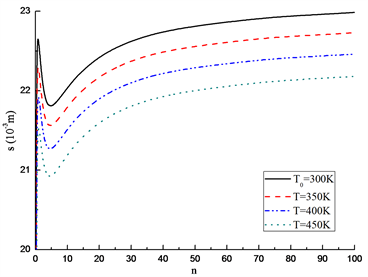(b) C-S边界(Clamped-Simply supported boundary)

Figure 5. Effect of volume fraction index n on elastoplastic interface s ( $L=10$ )

4. 结论

1) 基于Timoshenko剪切变形理论和辛方法研究了FGM梁的弹塑性屈曲，建立了Hamilton体系中的屈曲模态方程和分叉条件，通过解析求解获得了屈曲载荷和屈曲模态，同时也得到了弹塑性分界面。

2) 临界载荷随体积分数指数、环境温度和长细比的增大而减小。

3) FGM梁弹塑性屈曲时的塑性流动域受到梯度参数、长细比和边界条件的影响。对于较小长细比的FGM梁，弹塑性屈曲时屈服界面靠近陶瓷侧，塑性流动区较大；对于较大长细比的FGM梁，屈服界面更偏向金属侧，塑性流动区较小。

NOTES

*通讯作者。

 孙云, 陈江, 李世荣. 基于物理中面FGM板屈曲的有限元分析[J]. 计算力学学报, 2020, 37(5): 47-53.

 Shariyat, M. (2008) Dynamic Thermal Buckling of Suddenly Heated Temperature Dependent FGM Cy-lindrical Shells under Combined Axial Compression and External Pressure. International Journal of Solids and Structures, 45, 2598-2612.
https://doi.org/10.1016/j.ijsolstr.2007.12.015

 Li, S.R., Zhang, J.H. and Zhao, Y.G. (2007) Nonlinear Ther-momechanical Post Buckling of Circular FGM Plate with Geometric Imperfection. Thin-Walled Structures, 45, 528-536.
https://doi.org/10.1016/j.tws.2007.04.002

 Zhang, J.H., Pan, S.C. and Chen, L.K. (2019) Dynamic Thermal Buckling and Postbuckling of Clamped-Clamped Imperfect Functionally Graded Annular Plates. Nonlinear Dynamics, 95, 565-577.
https://doi.org/10.1007/s11071-018-4583-5

 Li, S.R., Zhang, J.H. and Zhao, Y.G. (2006) Thermal Post-Buckling of Functionally Graded Material Timoshenko Beams. Applied Mathematics and Mechanics, 27, 803-810.
https://doi.org/10.1007/s10483-006-0611-y

 Shen, H.S. (2007) Thermal Postbuckling of Shear Deformable FGM Cylindrical Shells with Temperature-Dependent Properties. Mechanics of Composite Materials and Structures, 14, 439-452.
https://doi.org/10.1080/15376490701298942

 Shen, H.S. (2007) Thermal Postbuckling Behavior of Shear Deformable FGM Plates with Temperature-Dependent Properties. International Journal of Mechanical Sciences, 49, 466-478.
https://doi.org/10.1016/j.ijmecsci.2006.09.011

 Kiani, Y. and Eslam, M.R. (2010) Thermal Buckling Analysis of Functionally Graded Material Beams. International Journal of Mechanics and Materials in Design, 6, 229-238.
https://doi.org/10.1007/s10999-010-9132-4

 Alijani, A., Darvizeh, M. and Darvizeh, A. (2015) Elastoplastic Pre- and Post-Buckling Analysis of Functionally Graded Beams under Mechanical Loading. Proceedings of the Institution of Mechanical Engineers, 229, 146-165.
https://doi.org/10.1177/1464420713502908

 Zhang, J. and Zheng, W. (2021) Elastoplastic Buckling of FGM Beams in Thermal Environment. Continuum Mechanics and Thermodynamics, 33, 151-161.
https://doi.org/10.1007/s00161-020-00895-z

 Beikmohammadlou, H. and Ekhteraeitoussi, H. (2017) Para-metric Studies on Elastoplastic Buckling of Rectangular FGM Thin Plates. Aerospace Science and Technology, 69, 513-525.
https://doi.org/10.1016/j.ast.2017.07.015

 Xu, G., Huang, H. and Chen, B. (2017) Buckling and Postbuckling of Elastoplastic FGM Plates under Inplane Loads. Composite Structures, 176, 225-233.
https://doi.org/10.1016/j.compstruct.2017.04.061

 Tsiatas, G.C. and Babouskos, N.G. (2017) Elastic-Plastic Analysis of Functionally Graded Bars under Torsional Loading. Composite Structures, 176, 254-267.
https://doi.org/10.1016/j.compstruct.2017.05.044

 Xu, G., Huang, H.W. and Han, Q. (2017) Study on Post-buckling of Axial Compressed Elastoplastic Functionally Graded Cylindrical Shells. Mechanics of Advanced Materials & Structures, 25, 820-828.
https://doi.org/10.1080/15376494.2017.1308589

 Zhang, Y., Huang, H. and Han, Q. (2015) Buckling of Elastoplastic Functionally Graded Cylindrical Shells under Combined Compression and Pressure. Composites Part B Engineering, 69, 120-126.
https://doi.org/10.1016/j.compositesb.2014.09.024

 Nemat-alla, M., Ahmed, K. and Hassab-allah, I. (2009) Elastic-Plastic Analysis of Two-Dimensional Functionally Graded Materials under Thermal Loading. International Journal of Solids and Structures, 46, 2774-2786.
https://doi.org/10.1016/j.ijsolstr.2009.03.008

 Reddy, J.N. and Chin, C.D. (1998) Thermomechanical Analysis of Functionally Graded Cylinders and Plates. Journal of Thermal Stresses, 21, 593-620.
https://doi.org/10.1080/01495739808956165

Top